# NCERT Solutions for Class 8 Math Chapter 10 - Visualising Solid Shapes

##### Question 1:

Match the following.1. $\to$ (ii) $\to$ (c)
2. $\to$ (v) $\to$ (g)
3. $\to$ (iii) $\to$ (b)
4. $\to$ (vii) $\to$ (h)
5. $\to$ (viii) $\to$ (f)
6. $\to$ (iv) $\to$ (d)
7. $\to$ (vi) $\to$ (e)
8. $\to$ (i) $\to$ (a)

##### Question 2:

Match the following pictures (objects) with their shapes: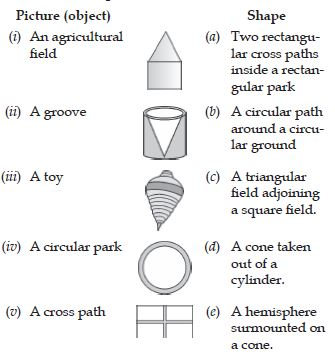(i) $\to$ (c) (ii) $\to$ (c) (iii) $\to$ (e)
(iv) $\to$ (b) (v) $\to$ (a)

##### Question 3:

For each of the given solid, the two views are given. Match for each solid the corresponding top and front views. The first one is done for you.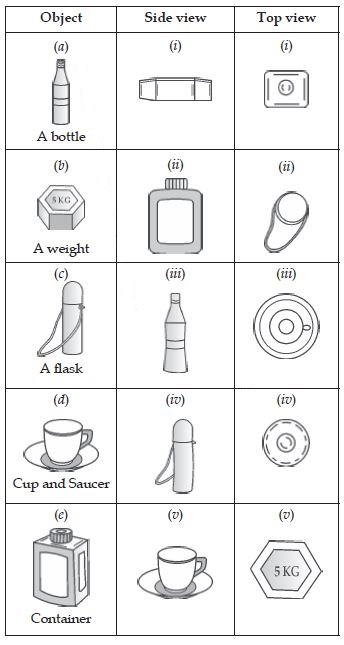(a) $\to$ (iii) $\to$ (iv)
(b) $\to$ (i) $\to$ (v)
(c) $\to$ (iv) $\to$ (ii)
(d) $\to$ (v) $\to$ (iii)
(e) $\to$ (ii) $\to$ (i)

##### Question 4:

For each of the given solid, the three views are given. Identify for each solid the corresponding top, front and side views.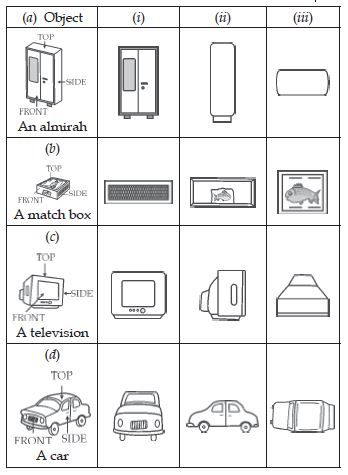(a) (i) Front (ii) Side (iii) Top
(b) (i) Side (ii) Front (iii) Top.
(c) (i) Front (ii) Side (iii) Top.
(d) (i) Front (ii) Side (iii) Top.

##### Question 5:

For each given solid, identify the top view, front view and side view.(a) (i) Top (ii) Front (iii) Side.
(b) (i) Side (ii) Front (iii) Top.
(c) (i) Top (ii) Side (iii) Front.
(d) (i) Side (ii) Front (iii) Top.
(e) (i) Front (ii) Top (iii) Side.

##### Question 6:

Draw the front view, side view and top view of the given objects.
A military tent
A table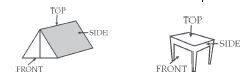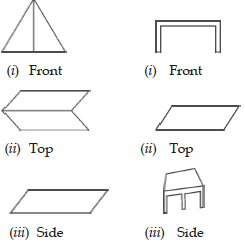##### Question 7:

Draw the front view, side view and top view of the given objects.
A nut
A hexagonal block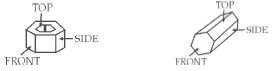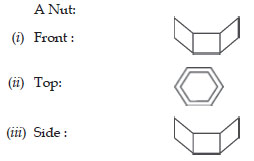##### Question 8:

Draw the front view, side view and top view of the given objects.
A dice
A solid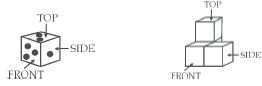##### Question 9:What happens to F, V and E if some parts are sliced off from a solid?

##### Question 10:

Can a polyhedron have for its faces:
(i) 3 triangles?
(ii) 4 triangles?
(iii) a square and four triangles?

(i) No
(ii) Yes, Pyramid
(iii) Yes, Square Pyramid.

##### Question 11:

Is it possible to have a polyhedron with any given number of faces?

Yes, Pyramid.

##### Question 12:

Which are Prism’s among the following: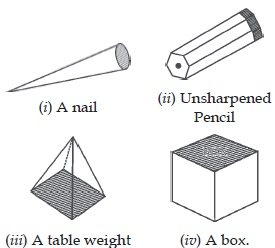(ii) Unsharpened Pencil and (iii) a table weight.

##### Question 13:

(i) How are prisms and cylinders alike?
(ii) How are pyramids and a cones alike?

(i) Both prism and cylinder may have common vertical axis.
(ii) Both pyramid and cone have only one vertex above the plane base.

##### Question 14:

Is a square prism same as a cube? Explain.

Yes, a square prism can be same as cube in the same way as a rectangular prism is same as cuboid.

##### Question 15:

Verify Euler’s formula for these solids: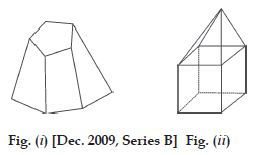(i) Number of faces F = 5
Number of vertices V = 9
Number of edges E = 12
$\therefore$ By Euler’s law :
F + V – E = 2
5 + 9 – 12 = 2
2 = 2
(ii) Number of faces F = 10
Number of vertices V = 9
Number of edges E = 17
$\therefore$ By Euler’s law :
F + V – E
10 + 9 – 17 = 2
2 = 2

##### Question 16:

Using Euler’s formula, find the unknown: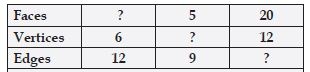According to Euler’s Formula :
F + V = E + 2
Therefore, we have: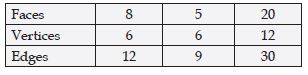##### Question 17:

Can a polyhedron have 10 faces, 20 edges and 15 vertices ?

$⇒$ 10 + 15 – 20
$⇒$ 25 – 20 = 5 $\ne$ 2.